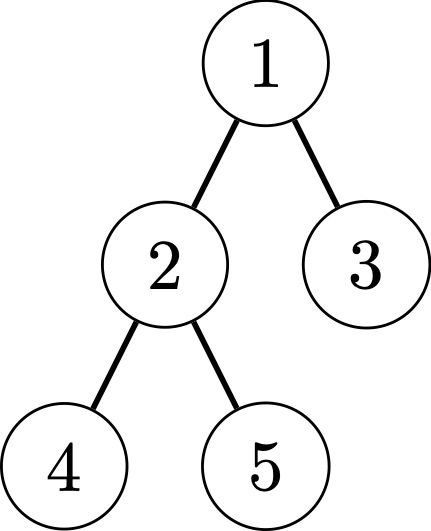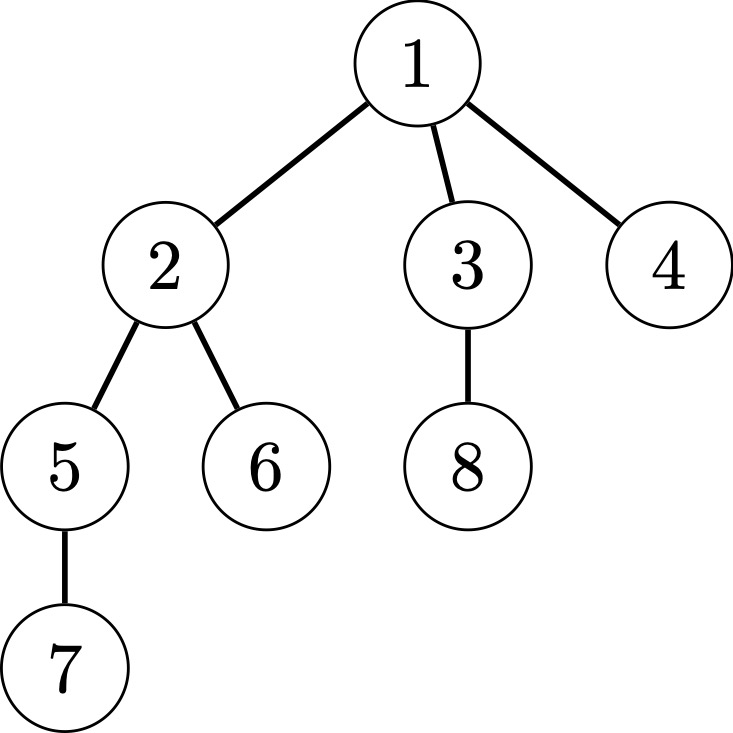시간 제한메모리 제한제출정답맞힌 사람정답 비율
2 초 (추가 시간 없음) 1024 MB111100.000%

## 문제

After finishing the IODS team selection, you want to travel to a new country to relax. The country contains $n$ cities, which are connected by $n-1$ bidirectional roads. There is a unique simple path between any two cities.

In the next $m$ days, you wish to explore the country. On each day you have set $k$ cities $x_1, x_2, \ldots, x_{k}$ that you wish to visit in order. At the beginning of each day, you can choose any city as the starting city of your trip, and then you need to reach city $x_1$, then city $x_2$, ..., and finally city $x_k$ to complete your day's travel.

To add to the fun of the trip, you don't want to pass through a city more than once in a day. At any given moment, you can choose to follow a road from this city to another city, or choose to take a yacht to any city.

You want to know, for each day of travel, the minimum number of yacht rides required in order to avoid passing through the same city more than once. Note that each day's travel is independent: a city passed on the previous day can still be passed on the next day.

## 입력

The first line contains two integers $n$ and $m$ ($1 \le n \le 2 \cdot 10^5$, $0 \le m \le 5 \cdot 10^4$).

Each of the next $n-1$ lines contains two integers $x$ and $y$ ($1 \le x,y \le n$, $x \ne y$), indicating that there is a bidirectional edge between vertices $x$ and $y$. It is guaranteed that the given graph is connected.

Each of the next $m$ lines describes queries in the format $k x_1 x_2 \ldots x_k$. It is guaranteed that $1 \le x_i \le n$ and $x_i \ne x_j$ for all $1 \le i < j \le k$.

The sum of $k$ in one test case does not exceed $2 \cdot 10^5$.

## 출력

For each day, output a single line containing a single integer: the minimum number of yacht rides.

## 예제 입력 1

5 3
1 2
1 3
2 4
2 5
3 1 4 5
4 1 2 4 3
4 2 4 5 1


## 예제 출력 1

1
1
2


## 예제 입력 2

8 7
1 2
1 3
1 4
2 5
2 6
5 7
3 8
1 4
2 1 7
3 5 2 4
4 3 6 1 4
6 5 3 7 1 2 4
6 4 8 3 5 6 1
7 2 8 5 4 6 1 3


## 예제 출력 2

0
0
0
1
4
3
5


## 힌트The figure corresponds to the first sample test caseThe figure corresponds to the second sample test case